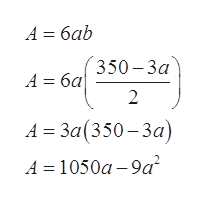# A farmer needs to take a rectangular plot of land and divide it into 6 fields of equal area. He has 700 feet of fence available to divide up this plot of land. What will be the dimensions for one of the fields if he wants to maximize the total area of the 6 fields?

Question
50 views

A farmer needs to take a rectangular plot of land and divide it into 6 fields of equal area. He has 700 feet of fence available to divide up this plot of land. What will be the dimensions for one of the fields if he wants to maximize the total area of the 6 fields?

check_circle

Step 1

Let a and b are the dimensions of each field as shown in the below figure.

Thus, the area of each field is a × b.

Therefore, the dimensions of the rectangular plot are 3a and 2b.

Let A be the area of the rectangular plot then we have

A = 6ab

Step 2

The perimeter of the rectangular plot is 2(2b + 3a)

Since the total fence available is 700 feet therefore the perimeter of the rectangular plot must be equals to the 700.

Step 3

Substituting the value of b in the expre...help_outlineImage TranscriptioncloseА -D баb 350-За А— ба 2 А - За 350-За ) А 3D 1050а -9a? fullscreen

### Want to see the full answer?

See Solution

#### Want to see this answer and more?

Solutions are written by subject experts who are available 24/7. Questions are typically answered within 1 hour.*

See Solution
*Response times may vary by subject and question.
Tagged in

### Derivative